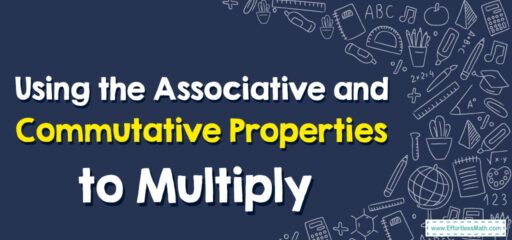# How to Use the Associative and Commutative Properties to Multiply

The associative and commutative properties are mathematical properties that allow us to manipulate the order of operations or the grouping of numbers without changing the result.## A Step-by-step Guide to Using the Associative and Commutative Properties to Multiply

Here’s a step-by-step guide on how to use the associative and commutative properties to multiply numbers:

### Step 1: Understand the properties

Familiarize yourself with the associative and commutative properties of multiplication. The commutative property states that the order of numbers in multiplication can be changed, while the associative property allows you to change the grouping of numbers without altering the result.

### Step 2: Identify the multiplication expression

Identify the multiplication expression you want to simplify using the properties. For example, let’s use the expression $$(2 x 3) x (4 x 5)$$.

### Step 3: Apply the associative property

Using the associative property, you can change the grouping of numbers. In this case, you can choose to group the numbers differently. Let’s group them as follows: $$(2 x 3) x (4 x 5) = 2 x (3 x 4) x 5$$

### Step 4: Apply the commutative property

Using the commutative property, you can change the order of multiplication. In this case, you can rearrange the numbers within each group. Let’s swap 3 and 4 within the parentheses: $$2 x (3 x 4) x 5 = 2 x (4 x 3) x 5$$

### Step 5: Simplify the expression

Perform the multiplication within the parentheses and evaluate the expression: $$2 x (4 x 3) x 5 = 2 x 12 x 5 = 24 x 5 = 120$$

### Step 6: Final result

The simplified expression is equal to 120. Therefore, $$(2 x 3) x (4 x 5)$$ is equal to 120.

By following these steps, you can effectively use the associative and commutative properties to simplify multiplication expressions. Remember that these properties can be applied in different orders, depending on what makes the calculation easier for you.

### What people say about "How to Use the Associative and Commutative Properties to Multiply - Effortless Math: We Help Students Learn to LOVE Mathematics"?

No one replied yet.

X
30% OFF

Limited time only!

Save Over 30%

SAVE $5 It was$16.99 now it is \$11.99